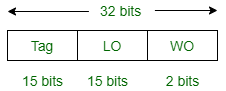# ISRO | ISRO CS 2020 | Question 31

• Last Updated : 04 Sep, 2020

How many total bits are required for a direct-mapped cache with 128 KB of data and 1 word block size, assuming a 32-bit address and 1 word size of 4 bytes ?
(A) 2 Mbits
(B) 1.7 Mbits
(C) 2.5 Mbits
(D) 1.5 Mbits

Explanation: Given,

```Data cache = 128 KB
Block size = 1 W = 4 Bytes
MM Address = 32 bit ```So, Number of lines,

```= CM Site / Block Size
= 128 KB / 4 B
= 32 K
= 215 ```

So, Tag memory size

```= Number of lines in cm × Tag space in the line
= 215 × 15 bit ```

Therefore, Total cache

```= Tag memory + Data memory
= 215 × 15 bit + 128 KB
= 215 × 15 bit + 128 K * 8 bits
= 480 K bit + 1024 K bit
= 1504 K bit
= 1.5 M bit ```

Option (D) is correct.

Quiz of this Question

My Personal Notes arrow_drop_up